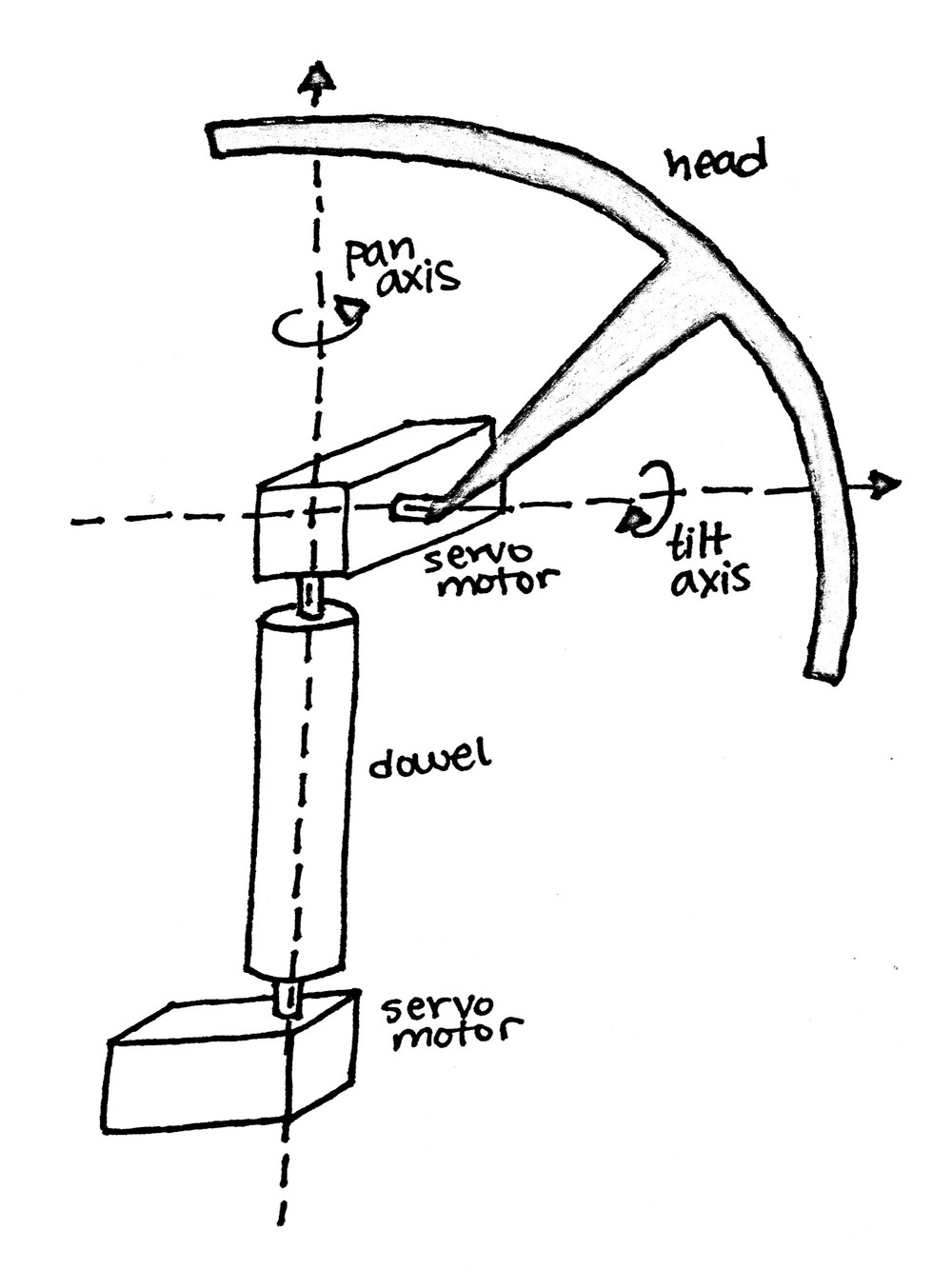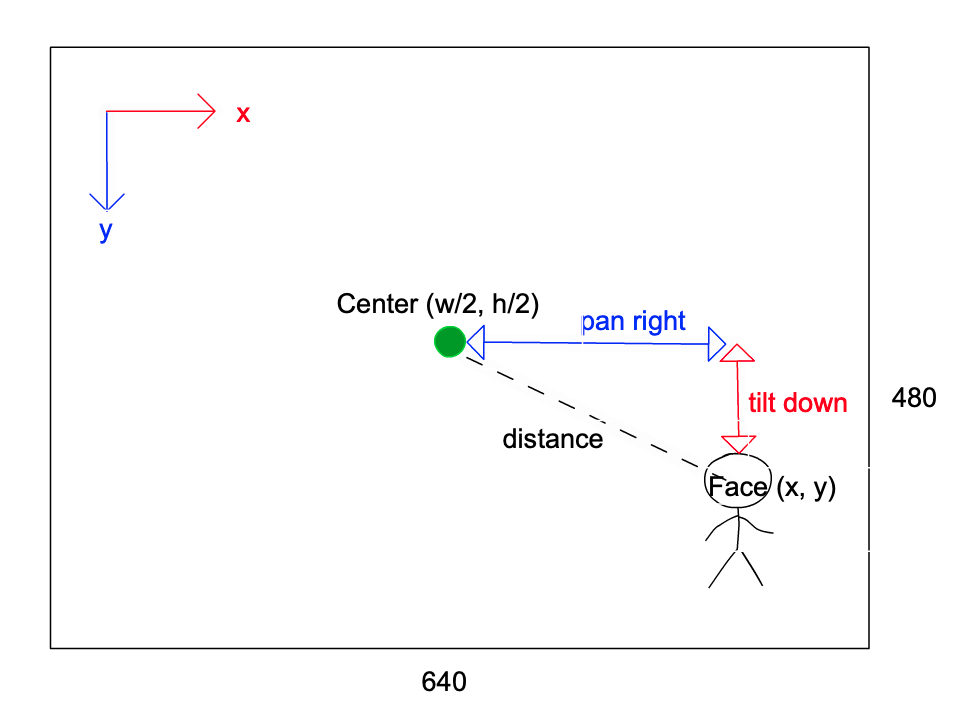# 基于 face_recognition 和 PID 的舵机云台人脸识别和跟踪

• pan: 水平左右方向转头
• tilt: 竖直上下方向转头## PID 控制

$u(t)=K_{\mathrm{p}} e(t)+K_{\mathrm{i}} \int_{0}^{t} e\left(t^{\prime}\right) d t^{\prime}+K_{\mathrm{d}} \frac{d e(t)}{d t}$

• 时间 $t$，在这里时间是离散的；
• 偏差 $e(t)$，在人脸跟踪中指的是图像中心与人脸中心之间的距离（x 方向，y 方向）；
• 系统输出 $u(t)$，即输出的舵机角度(分水平和垂直方向两个舵机的角度)；

### 比例(P)

• 当人脸与图像中心相距较远时，需要舵机大幅度运动对准人脸
• 当人脸与图像中心相距较近时，需要舵机小幅度靠近对准人脸

## 代码设计

• $x$ 轴偏差 对应 水平左右转动 pan
• $y$ 轴偏差 对应 垂直上下转动 tilt

• 受限于设备的性能，使用 face_recognition 识别一帧图像里的人脸可能会非常耗时
• 舵机控制时，不同的舵机响应时间不同
• PID 在代码中是通过循环累加来计算的；